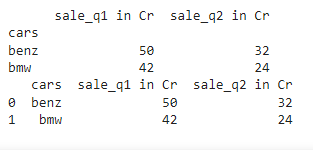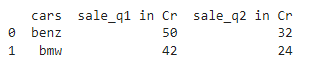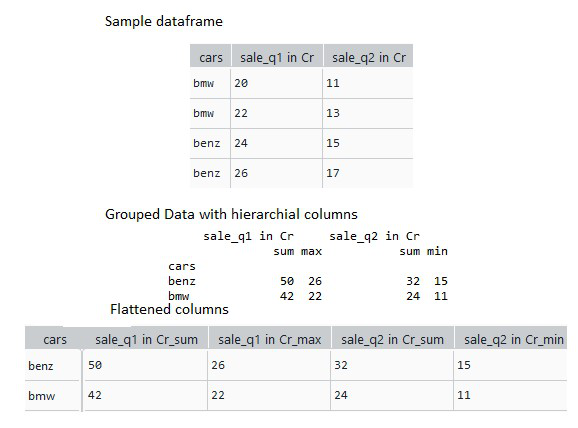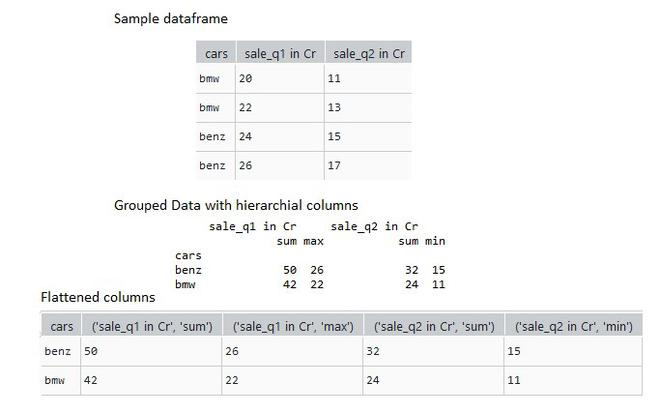Open In App

# How to flatten a hierarchical index in Pandas DataFrame columns?

In this article, we are going to see the flatten a hierarchical index in Pandas DataFrame columns. Hierarchical Index usually occurs as a result of groupby() aggregation functions. Flatten hierarchical index in Pandas, the aggregated function used will appear in the hierarchical index of the resulting dataframe.

## Using reset_index() function

Pandas provide a function called reset_index() to flatten the hierarchical index created due to the groupby aggregation function in Python

Syntax: pandas.DataFrame.reset_index(level, drop, inplace)

Parameters:

• level – removes only the specified levels from the index
• drop – resets the index to the default integer index
• inplace – modifies the dataframe object permanently without creating a copy.

Example:

In this example, We used the pandas groupby function to group car sales data by quarters and reset_index() pandas function to flatten the hierarchical indexed columns of the grouped dataframe.

## Python3

 `# import the python pandas package``import` `pandas as pd` `# create a sample dataframe``data ``=` `pd.DataFrame({``"cars"``: [``"bmw"``, ``"bmw"``, ``"benz"``, ``"benz"``],``                     ``"sale_q1 in Cr"``: [``20``, ``22``, ``24``, ``26``],``                     ``'sale_q2 in Cr'``: [``11``, ``13``, ``15``, ``17``]},``                    ` `                    ``columns``=``[``"cars"``, ``"sale_q1 in Cr"``,``                             ``'sale_q2 in Cr'``])` `# group by cars based on the sum``# of sales on quarter 1 and 2``grouped_data ``=` `data.groupby(by``=``"cars"``).agg(``"sum"``)` `print``(grouped_data)` `# use  reset_index to flattened``# the hierarchical dataframe.``flat_data ``=` `grouped_data.reset_index()` `print``(flat_data)`

Output:How to flatten a hierarchical index in Pandas

## Using as_index() function

Pandas provide a function called as_index() which is specified by a boolean value. The as_index() functions groups the dataframe by the specified aggregate function and if  as_index() value is False, the resulting dataframe is flattened.

Syntax: pandas.DataFrame.groupby(by, level, axis, as_index)

Parameters:

• by – specifies the columns on which the groupby operation has to be performed
• level – specifies the index at which the columns has to be grouped
• axis – specifies whether to split along rows (0) or columns (1)
• as_index – Returns an object with group labels as the index, for aggregated output.

Example:

In this example,  We are using the pandas groupby function to group car sales data by quarters and mention the as_index parameter as False and specify the as_index parameter as false ensures that the hierarchical index of the grouped dataframe is flattened.

## Python3

 `# group by cars based on the``# sum of sales on quarter 1 and 2``# and mention as_index is False``grouped_data ``=` `data.groupby(by``=``"cars"``, as_index``=``False``).agg(``"sum"``)` `# display``print``(grouped_data)`

Output:How to flatten a hierarchical index in Pandas

## Flattening hierarchical index in pandas dataframe using groupby

Whenever we use the groupby function on a single column with multiple aggregation functions we get multiple hierarchical indexes based on the aggregation type. In such cases, the hierarchical index has to be flattened at both levels.

Syntax: pandas.DataFrame.groupby(by=None, axis=0, level=None)

Parameter:

• by – mapping function that determines the groups in groupby function
• axis – 0 – splits along rows and 1 – splits along columns.
• level – if the axis is multi-indexed, groups at a specified level. (int)

Syntax: pandas.DataFrame.agg(func=None, axis=0)

Parameter:

• func – specifies the function to be used as aggregation function. (min, max, sum etc)
• axis – 0 – function applied to each column and 1- applied to each row.

Example

Import the python pandas package. Create a sample dataframe showing the car sales in two-quarters q1 and q2 as shown. Now use the pandas groupby function to group based on the sum and max of sales on quarter 1 and sum and min of sales 2. The grouped dataframe has multi-indexed columns stored in a list of tuples. Use a for loop to iterate through the list of tuples and join them as a single string. Append the joined strings in the flat_cols list. </li > <li > Now assign the flat_cols list to the column names of the multi-indexed grouped dataframe columns.

## Python3

 `# group by cars based on``# the sum and max of sales on quarter 1``# and sum``grouped_data ``=` `data.groupby(by``=``"cars"``).agg(``                 ``{``"sale_q1 in Cr"``: [``sum``, ``max``],``                ``'sale_q2 in Cr'``: [``sum``, ``min``]})` `# create an empty list to save the``# names of the flattened columns``flat_cols ``=` `[]` `# iterate through this tuples and``# join them as single string``for` `i ``in` `grouped_data.columns:``    ``flat_cols.append(i[``0``]``+``'_'``+``i[``1``])` `# now assign the list of flattened``# columns to the grouped columns.``grouped_data.columns ``=` `flat_cols` `# print the grouped data``print``(grouped_data)`

Output:How to flatten a hierarchical index in Pandas

## Flattening hierarchical index using to_records() function

In this example, we use the to_records() function of the pandas dataframe which converts all the rows in the dataframe as an array of tuples. This array of tuples is then passed to pandas.DataFrame function to convert the hierarchical index as flattened columns.

Syntax: pandas.DataFrame.to_records(index=True, column_dtypes=None)

Explanation:

• index – creates an index in resulting array
• column_dtypes – sets the columns to specified datatype.

Code:

## Python3

 `# group by cars based on the sum``# and max of sales on quarter 1``# and sum and min of sales 2 and mention``# as_index is False``grouped_data ``=` `data.groupby(by``=``"cars"``).agg({``"sale_q1 in Cr"``: [``sum``, ``max``],``                                            ``'sale_q2 in Cr'``: [``sum``, ``min``]})``# use to_records function on grouped data``# and pass this to the Dataframe function``flattened_data ``=` `pd.DataFrame(grouped_data.to_records())` `print``(flattened_data)`

Output:How to flatten a hierarchical index in Pandas

## Flattening hierarchical columns using join() and rstrip()

In this example, we use the join() and rstrip() functions to flatten the columns. Usually, when we group a dataframe as hierarchical indexed columns, the columns at multilevel are stored as an array of tuples elements.

Syntax: str.join(iterable)

Explanation: Returns a concatenated string, if iterable, else returns a type error.

Syntax: str.rstrip([chars])

Explanation: Returns a string by splitting the excess trailing spaces (rightmost) to the string.

Code:

Here, we iterate through these tuples by joining the column name and index name of each tuple and storing the resulting flattened columns name in a list. Later, this stored list of flattened columns is assigned to the grouped dataframe.

## Python3

 `# group by cars based on the sum``# and max of sales on quarter 1``# and sum and min of sales 2 and``# mention as_index is False``grouped_data ``=` `data.groupby(by``=``"cars"``).agg({``"sale_q1 in Cr"``: [``sum``, ``max``],``                                            ``'sale_q2 in Cr'``: [``sum``, ``min``]})` `# use join() and rstrip() function to``# flatten the hierarchical columns``grouped_data.columns ``=` `[``'_'``.join(i).rstrip(``'_'``)``                        ``for` `i ``in` `grouped_data.columns.values]` `print``(grouped_data)`

Output:How to flatten a hierarchical index in Pandas